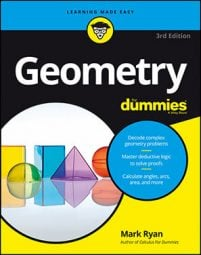##### Geometry For DummiesPyramids are full of congruent right triangles. So, to find the volume of a pyramid, it's just a matter of using the Pythagorean Theorem or your knowledge of special right triangles.

A pyramid is a solid figure with a polygonal base and edges that extend up from the base to meet at a single point. The corners of a pyramid are called vertices, the segments that connect the vertices are called edges, and the flat sides are called faces.

A regular pyramid is a pyramid with a regular-polygon base, whose peak is directly above the center of its base. The lateral faces of a regular pyramid are all congruent. Most pyramids in geometry books are regular pyramids.

Here's how to find the volume of a pyramid.

Now, let's apply it to a pyramid problem.

Now here's the proof diagram.

To compute the volume of a pyramid, you need its height (line AZ) and the area of its square base, PRTV. You can get the height by solving right triangle PZA. The lateral edges of a regular pyramid are congruent; thus, the hypotenuse of triangle PZA, line PZ, is congruent to line RZ, so its length is also 10. Line PA is half of the diagonal of the base, so it's 6. Triangle PZA is thus a 3-4-5 triangle blown up to twice its size, namely a 6-8-10 triangle, so the height, line AZ, is 8 (or you can use the Pythagorean Theorem to get line AZ).

To get the area of square PRTV, you can, of course, first figure the length of its sides; but don't forget that a square is a kite, so you can use the kite area formula instead—that's the quickest way to get the area of a square if you know the length of a diagonal. Because the diagonals of a square are equal, both of them are 12, and you have what you need to use the kite area formula:

Now use the volume formula: